I took a couple of evening to look how TV remotes works and how can we simply interface them with our PC (with minimal hw requirements) and I’d like to share the results with you.

First of all we will need a way to capture those infrared signal coming from our remotes, the easiest way (and cheapest) is to build a small receiver with a photo-diode. (on the sides you see the electric diagram and my test bench)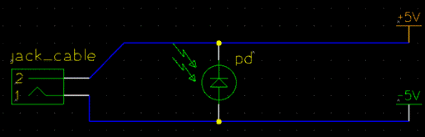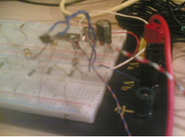Now that we built our receiver we can test if it’s working like it should. I used Audacity (a Linux audio multifunctional tool) to analyze the signal coming from the photodiode. The results: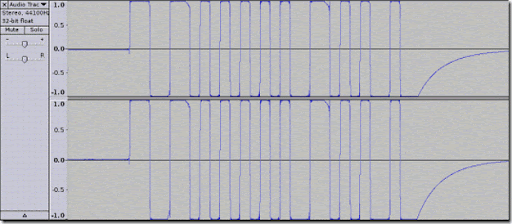Now we have a signal composed by some waves, great, let’s try to explain how CIR (Consumer infrared) works.
First of all, quite each brand has his own solution, so we can’t really generalize the discussion. Anyway the most used is the RC5 from Philips. As you can remember, the lesser the frequency the the fair signal can get. The modulation (how you encode your data on the wave) used by fast every brand is called Amplitude-Shift Keying (ASK).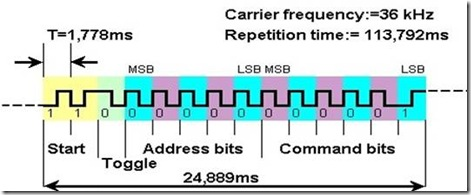RC5 Structure:
Each frame is composed by:
1. 2 start bits which are used by the AGC (Automatic Gain Controller) from the receiver (TV)
2. 1 toggle bit which varies every time a button is pressed
3. 5 bits which represents the device address
4. 6 bits with the command
Bits are encoded with Manchester format, for this reason a logical zero is in practice a 1-0 and a logical one (1) is a 0-1, you can probably better understand this by the following schema: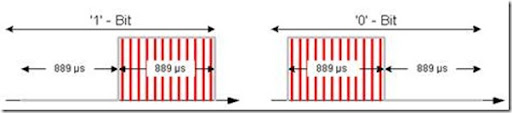Now that we have a minimal understanding of the ground let’s try code something with this. First of all we have to access our audio mic line (where we connected our fancy IR receiver) and prepare a buffer that our system will fill up with sampled data. Fortunately Linux provide a simple way to capture data from your soundcard.
`   1: void init_audio(void)`
`   2: {`
`   3:     int rc;`
`   4:     int val, dir;`
`   5:  `
`   6:     // Open PCM device for capture `
`   7:     rc = snd_pcm_open(&handle, "default",  SND_PCM_STREAM_CAPTURE, 0);`
`   8:     if (rc < 0) {`
`   9:         fprintf(stderr,`
`  10:                 "Unable to open pcm device: %s\n",`
`  11:                 snd_strerror(rc));`
`  12:         exit(1);`
`  13:     }`
`  14:     `
`  15:     // Setting default samples `
`  16:     snd_pcm_hw_params_alloca(&params);`
`  17:     snd_pcm_hw_params_any(handle, params);`
`  18:     snd_pcm_hw_params_set_access(handle, params, SND_PCM_ACCESS_RW_INTERLEAVED);`
`  19:     `
`  20:     // 8bits Signed samples `
`  21:     snd_pcm_hw_params_set_format(handle, params, SND_PCM_FORMAT_S8);`
`  22:     `
`  23:     // Single channel (mono) `
`  24:     snd_pcm_hw_params_set_channels(handle, params, 1);`
`  25:     `
`  26:     // Setting sampling rate `
`  27:     val = SAMPLERATE;`
`  28:     snd_pcm_hw_params_set_rate_near(handle, params,`
`  29:                                     &val, &dir);`
`  30:     `
`  31:     // Set period size to 300 frames. `
`  32:     frames = 300;`
`  33:     snd_pcm_hw_params_set_period_size_near(handle,   params, &frames, &dir);`
`  34:     `
`  35:     // Write the parameters to the driver `
`  36:     rc = snd_pcm_hw_params(handle, params);`
`  37:  `
`  38:     if (rc < 0) {`
`  39:         fprintf(stderr,`
`  40:                 "Unable to set hw parameters: %s\n",`
`  41:                 snd_strerror(rc));`
`  42:         exit(1);`
`  43:     }`
`  44:     `
`  45:     // Use a buffer large enough to hold one period `
`  46:     snd_pcm_hw_params_get_period_size(params,  &frames, &dir);`
`  47:     size = frames * 1; // 1 bytes/sample, 1 channels `
`  48:  `
`  49:     buffer = (char *) malloc(size);`
`  50:     `
`  51:     // We want to loop for 2 seconds `
`  52:     snd_pcm_hw_params_get_period_time(params,`
`  53:                                       &val, &dir);    `
`  54:     `
`  55:     printf("Audio initialized!\n");`
`  56: }`
`  57:  `
`  58: // listen function used as a dedicated thread (pthread_create())`
`  59: void *listen_function(void)`
`  60: {`
`  61:     int rc;`
`  62:     int j;`
`  63:     `
`  64:     while (!QUIT) {`
`  65:         // variable has been initialized in init_audio function`
`  66:         rc = snd_pcm_readi(handle, buffer, frames); // read "frames" from soundcard`
`  67:  `
`  68:         if (rc == -EPIPE) {`
`  69:             // EPIPE means overrun `
`  70:             fprintf(stderr, "overrun occurred\n");`
`  71:             snd_pcm_prepare(handle);`
`  72:             `
`  73:         } else if (rc < 0) {`
`  74:             fprintf(stderr,    "error from read: %s\n", snd_strerror(rc));`
`  75:         }`
`  76:  `
`  77:         // draw signal to stdout`
`  78:         //graph(buffer, rc);`
`  79:  `
`  80:         if(DEBUG)`
`  81:         {`
`  82:             for(j=0; j<rc;j++){`
`  83:                 fprintf(fp, "%.1f\t%d\n", (double)(delta*1000.0*(double)counter), buffer[j]);`
`  84:                 counter++;`
`  85:             }`
`  86:         }`
`  87:         // elaborate buffer`
`  88:         computeDelta(buffer,rc);`
`  89:     }`
`  90:     printf("ListenThread terminated!\n");`
`  91:     closeAudio();`
`  92:     pthread_exit(NULL);`
`  93: }`
Next we need a simple algorithm to discriminate the length of the peaks we read from the photodiode:
`   1: #define SAMPLERATE 44100`
`   2: #define DELTATHRESHOLD 0.5`
`   3: #define TRUE 1`
`   4: #define FALSE 0`
`   5: #define THRESOLDLIMIT 6 // Under this no calculation is made [range 1-127]`
`   6: #define DEBUG 0  // If true: verbose output + sampled data written to logfile.txt`
`   7:  `
`   8: char OVERTH=FALSE; // FLAG used to know if signal is below or above threshold`
`   9: char PEAK = FALSE; // FLAG used to let know when a peak occurred`
`  10:  `
`  11: /* delta is time (in milliseconds) between each sample */`
`  12: double delta=(1.0/(double)SAMPLERATE*1000);`
`  13:  `
`  14: void computeDelta(char *buffer, int length)`
`  15: {`
`  16:     int k,j;`
`  17:     int maxgraph= -100;`
`  18:     int threshold;`
`  19:     int temp;`
`  20:  `
`  21:     int countstep=0;`
`  22:     double dpeak; // delta peak`
`  23:     `
`  24:     // Find max value in sampled buffer`
`  25:     for(j=0; j<length;j++)`
`  26:     {`
`  27:         if(buffer[j]>maxgraph)`
`  28:             maxgraph=buffer[j];`
`  29:     }`
`  30:     `
`  31:     // Compute threshold against max value`
`  32:     threshold = (int)((double)maxgraph*(double)DELTATHRESHOLD);`
`  33:     `
`  34:     // Check if signal rise above threshold`
`  35:     if(threshold > THRESOLDLIMIT)`
`  36:     {`
`  37:         if(DEBUG) printf("Threshold: %d [MAX: %d]\n", threshold, maxgraph);`
`  38:         for(k=0;k<length;k++)`
`  39:         {`
`  40:             // peak length`
`  41:             dpeak = ((double)(delta)*(double)countstep);`
`  42:             `
`  43:             // signal low (below 0) `
`  44:             if(buffer[k]>threshold && OVERTH == FALSE){`
`  45:                     if(DEBUG) printf("Low Peak length: %8.3f ms [Steps: %d][Value: %4d]\n", dpeak,countstep, buffer[k]);    `
`  46:                     // peak triggered when delta peak is longer than 0.4 ms                    `
`  47:                     if(dpeak>0.4)`
`  48:                         PEAK=TRUE;`
`  49:                     // reset counterstep for next peak`
`  50:                     countstep=0;`
`  51:                     countstep++;`
`  52:                     OVERTH = TRUE;`
`  53:             }`
`  54:             // signal high (above 0)`
`  55:             else if(buffer[k]<threshold && OVERTH==TRUE){`
`  56:                     if(DEBUG) printf("High Peak length: %8.3lf ms [Steps: %d][Value: %4d]\n", dpeak,countstep, buffer[k]);`
`  57:                     // peak triggered when delta peak is longer than 0.4 ms`
`  58:                     if(dpeak>0.4)`
`  59:                         PEAK=TRUE;`
`  60:                     // reset counterstep for next peak`
`  61:                     countstep=0;`
`  62:                     countstep++;`
`  63:                     OVERTH=FALSE;`
`  64:             }`
`  65:             // Peak detected`
`  66:             if(PEAK==TRUE)`
`  67:             {`
`  68:                 // I know the peak length should be within 800 us - 1.8 ms`
`  69:                 if(dpeak<2.0)`
`  70:                 {`
`  71:                     temp=(int)dpeak;`
`  72:                     // temp is one if peak is longer than 1 ms or zero if shorter`
`  73:                     cmd = (cmd<<1) | temp;`
`  74:                 }`
`  75:                 else`
`  76:                 {`
`  77:                     // command ready`
`  78:                     if(cmd > 0)`
`  79:                     {`
`  80:                         printf("CMD: %3d\n", cmd);`
`  81:                         cmd=0;`
`  82:                     }`
`  83:                 }`
`  84:                 PEAK = FALSE;`
`  85:             }`
`  86:             countstep++;`
`  87:         }`
`  88:     }`
`  89: }    `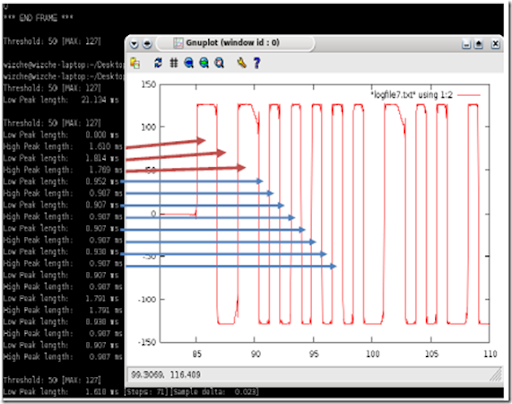The results are shown in the picture. Each peak is measured to see how long is it and  was that the shortest peaks (shrinked) have a length of around 900 us and the long peak a length of about 1.8 ms.
A simple proof of this values is done by comparing the graph (gnuplot) generated on the raw sampled data and the program result. If we compare the peak type (long/short) and the peak length we will see a “perfect” match.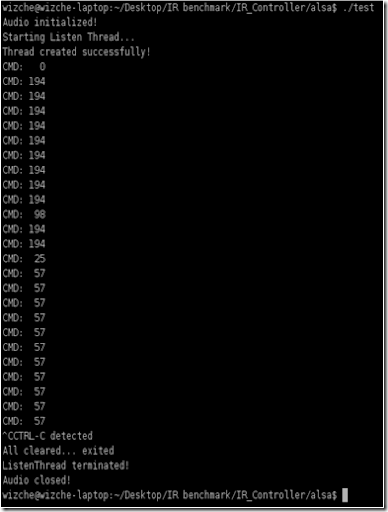Now we have do identify and differentiate the remote’s command (which button pressed).
A simple way to do this is using some bitwise operator. Let’s say that if the peak is longer than 1 ms we insert a one (bit) into our command (8 bit uchar), if shorter we insert a zero (bit).

If you take a look in the code above you will see that there is no length check when shifting and inserting new bit into command. therefore the algorithm will take only the last 8 peaks into the command (the other will be “overwritten”).

Trying the program will results in a series of command number that are associated to remote buttons. In the screenshot is shown the result of pressing two different buttons: channel – (CMD: 194), and volume + (CMD: 57) from a Philips remote.
The algorithm needs of course some more improvements since as you see the commands some time are wrong (0, 98, 25).

Now that we have a list of commands associated with buttons we could move the mouse respectfully with the remote you’re using:
`   1: if(cmd==62 || cmd==254)`
`   2: {`
`   3:     printf("Move 10 px left\n");`
`   4:     mouse_move(-10,0);`
`   5: }`
`   6: if(cmd==57 || cmd==249)`
`   7: {`
`   8:     printf("Move 10 px right\n");`
`   9:     mouse_move(10,0);`
`  10: }`
`  11: if(cmd==194)`
`  12: {`
`  13:     printf("Move 10 px down\n");`
`  14:     mouse_move(0,10);`
`  15: }`
`  16: if(cmd==192)`
`  17: {`
`  18:     printf("Move 10 px up\n");`
`  19:     mouse_move(0,-10);`
`  20: }`
`  21:  `
`  22: // Move mouse `
`  23: // Current Position + x / y`
`  24: void mouse_move(int x, int y)`
`  25: {`
`  26:         Display *displayMain = XOpenDisplay(NULL);`
`  27:  `
`  28:         if(displayMain == NULL)`
`  29:         {`
`  30:                 fprintf(stderr, "Error Opening Display!\n");`
`  31:                 exit(EXIT_FAILURE);`
`  32:         }`
`  33:  `
`  34:         XWarpPointer(displayMain, None, None, 0, 0, 0, 0, x, y);`
`  35:  `
`  36:         XCloseDisplay(displayMain);`
`  37: }`
Maybe it comes handy sometime to draw the signal direct into the console output, for this reason I’ve created a simple ascii graph function to display my buffer (sampled data) content.
`   1: // Simple ascii graph function`
`   2: // Draw char buffer to stdout using printf`
`   3: void graph(char *buffer, int length)`
`   4: {`
`   5:     `
`   6:     int iy;`
`   7:     char mingraph=126, maxgraph=-126;    `
`   8:     int temp;`
`   9:     unsigned int val;`
`  10:     int i,j,k;`
`  11:     `
`  12:     // Find max / min`
`  13:     for(j=0; j<length;j++)`
`  14:     {`
`  15:         if(buffer[j]>maxgraph)`
`  16:             maxgraph=buffer[j];`
`  17:         if(buffer[j]<mingraph)`
`  18:             mingraph=buffer[j];`
`  19:     }`
`  20:     `
`  21:     for(k=0;k<length;k++)`
`  22:     {`
`  23:         val=buffer[k];`
`  24:         if(mingraph<0) `
`  25:             val-=mingraph;`
`  26:         `
`  27:         iy=(val*maxgraph)/(maxgraph-mingraph);`
`  28:         printf("%4d ", val);`
`  29:         for(i=0;i<iy;i++) `
`  30:             printf(" ");         `
`  31:         printf("*\n"); `
`  32:     }`
`  33: }`
That’s it.